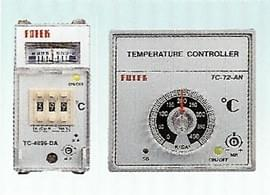# Temp. Controller-TC-72

More Details
Temperature Controller
TC-72 series
-General specification
1. High rellability by S. M. T. & TQC.
2. With cold junction compensation, suited to operated
at  -20
℃
~ + 60
3. All type with sensor break alarm LED.
4. With P+D control to control temperature easily.
5. High accuracy of displaying & setting less than 0.5%
of F. S.
6. With stand-by / over-heat alarm. (Optioned)
7. With heater break alarm. (Optioned)
-Purchase guide:
EX:
TC

72
-
D

A
-
PT
-

3
-
F
-
S
-
AH

(1) (2)   (3)(4)    (5)   (6)(7)    (8)  (9)  (10)
(1) Series
TC = Temperature Controller
(2) Outline (W)x(H)
Knockout
72 = 72 x 72 x 117       68 x 68
4896 = 48 x 96 x 100    45 x 92
(3) Method of Setting
A = Trimmer             D = Digital switch
(4) Method of Display
A = Analog deviation meter
D = Digital LED Display
N = Without display
(5) Type of Sensor
PT = PTD PT-100 W
J = J (IC) type thermocouple
Non = K (CA) type thermocouple
(6) Method of output
R = Relay (5A / 250 VAC)
V = Voltage (DC12V/50mA)
L = Linear (4~20mA)
(7) Range of Setting

Digital Switch

Trimmer
0 = -99 ~ +  99      05 = -50 ~ +50
1 =    0 ~ + 199       1 =     0 ~ +100
3 =    0 ~ + 399       2 =     0 ~ +200
5 =    0 ~ + 599       4 =     0 ~ +400
9 =    0 ~ + 999       6 =     0 ~ +600
11=    0 ~ +1199      12=     0 ~+1200
(8) Unit of Degree
F = oF            non = oC
(9) Method of Control
S = ON/OFF     non =P+D ON/OFF
(10) Alarm
A = With stand-by / Over heat alarm (AL)
AH = With stand-by / Over heat alarm
& Heater break alarm (AL + HB)